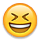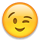# Algorithm to solve log puzzle

edited December 2010
Hey, dunno if anyone still visits this part of the forum, but thought you might like this nifty thing. A friend of mine was playing Puzzle Agent yesterday and decided to program something that would solve one of the latter log puzzles. As he said in the email:
I had to try a few different techniques until I found one efficient enough (there are 3^18 = 387,420,489 possibilities to try!). If you're interested, below is the (somewhat ugly) code that ended up finding the right solution instantly.

So he did it! Here's the code. You can run it your favourite C++ compiler and see this cool little program in action(Code posted with his permission.)
``````#include <iostream>
#include <set>
#include <algorithm>

using std::cout;
using std::endl;
using std::set;
using std::pair;
using std::make_pair;

/* Data types. */
typedef int Direction;
const Direction UP = 0;
const Direction DOWN = 1;
const Direction RIGHT = 2;
const Direction LEFT = 3;

typedef pair<int, int> Point;

struct State {
// Current position.
int x, y;
// The number of forward and backward slashes remaining.
int fwds, bwds;
// Current movement direction.
Direction dir;
// The coordinates of the stars collected so far.
set<Point> collected;
// The coordinate/direction pairs that we've passed so far. If we ever pass
// one such pair more than once, we're in an infinite loop.
set<pair<Point, Direction> > seen;
};

/* Global board, edited while trying solutions. */
char board[] = {
{' ', '/', '*', ' ', ' ', 'S'},
{'/', ' ', '*', '*', ' ', ' '},
{'*', '*', ' ', '*', '*', ' '},
{' ', '*', '*', '*', '*', ' '},
{' ', ' ', '*', '*', ' ', ' '},
{'D', ' ', ' ', ' ', ' ', '/'}
};

/* Prints out the board. */
void print() {
cout << endl;
for (int i=0; i<6; i++) {
for (int j=0; j<6; j++) {
cout << board[i][j];
}
cout << endl;
}
cout << endl;
}

/* Moves the coordinate x,y in direction d. */
void move(Direction d, int& x, int& y) {
if (d == UP) y--;
else if (d == DOWN) y++;
else if (d == RIGHT) x++;
else if (d == LEFT) x--;
}

/* Calculates the direction after bouncing from a given barried. */
Direction bounce(Direction d, char barrier) {
if (barrier == '/') {
if (d == UP) d = RIGHT;
else if (d == DOWN) d = LEFT;
else if (d == RIGHT) d = UP;
else if (d == LEFT) d = DOWN;
} else if (barrier == '\\') {
if (d == UP) d = LEFT;
else if (d == DOWN) d = RIGHT;
else if (d == RIGHT) d = DOWN;
else if (d == LEFT) d = UP;
} else {
// Invalid barrier. Crash and burn!
exit(1);
}
return d;
}

/* Checks whether the current board is solved. */
bool test() {
int stars_to_find = 0;
for (int i=0; i<6; i++) {
for (int j=0; j<6; j++) {
if (board[i][j] == '*') stars_to_find++;
}
}

State s;
s.x = 5;
s.y = 0;
s.dir = DOWN;

while (s.x >= 0 && s.x <= 5 && s.y >= 0 && s.y <= 5) {
pair<Point, Direction> cur = make_pair(make_pair(s.x, s.y), s.dir);
if (s.seen.count(cur)) break;
s.seen.insert(cur);

move(s.dir, s.x, s.y);

switch (board[s.y][s.x]) {
case 'D':
return s.collected.size() == stars_to_find;
case '*':
s.collected.insert(make_pair(s.x, s.y));
break;
case '/':
case '\\':
s.dir = bounce(s.dir, board[s.y][s.x]);
}
}

return false;
}

/* Traverses the board, splitting recursively on empty squares. */
void place(State s) {
while (s.x >= 0 && s.x <= 5 && s.y >= 0 && s.y <= 5) {
switch (board[s.y][s.x]) {
case 'S':
// Nothing to see here, move along.
break;
case 'D':
// Do a clean check. We have to do this because place() does not
// account for the effect of newly placed barriers on paths already
// traversed and may give false positives.
if (test()) {
cout << "Solution found:" << endl;
print();
exit(0);
}
return;
case '*':
s.collected.insert(make_pair(s.x, s.y));
break;
case '/':
case '\\':
s.dir = bounce(s.dir, board[s.y][s.x]);
break;
case ' ':
// Try filling the space with barriers if we have some available,
// recursively traversing the resulting board(s).
pair<Point, Direction> cur = make_pair(make_pair(s.x, s.y), s.dir);

if (s.fwds) {
State new_s = s;
new_s.seen.insert(cur);
new_s.fwds--;
board[s.y][s.x] = '/';
place(new_s);
}
if (s.bwds) {
State new_s = s;
new_s.seen.insert(cur);
new_s.bwds--;
board[s.y][s.x] = '\\';
place(new_s);
}

board[s.y][s.x] = ' ';
break;
}

move(s.dir, s.x, s.y);

if (s.seen.count(make_pair(make_pair(s.x, s.y), s.dir))) break;
}
}

int main() {
cout << "Starting map:" << endl;
print();

State s;
s.x = 5;
s.y = 0;
s.dir = DOWN;
s.fwds = 6;
s.bwds = 6;

place(s);

// place() exits if it finds a solution. If we've reached this, it failed.
cout << "No solution found." << endl;
}
``````

• edited December 2010
You are still trying 2,673,216 possibilities, I think you can improve that.

Just an idea:

Initialisation:
``````/ *     S
/   * *
* *   * *
* * * *
* *
D         /
x=5, y=0, 6 logs /, 6 logs \, Dir = down
``````
Path calculation:
``````/ *     S
/   * *   #
* *   * * #
* * * * #
* *   #
D#########/
``````
Solution not found, therefore, the first movable log encountered by the car must be somewhere in the sharps (#).

Loop the "sharp positions" try to replace them by either \ or /, recursion.

Example, I try to put a \ log in the (1,5) position. I assumed that it is the first log that the car will encounter. Therefore, there can't be an other log where a "S" stands. We move the car into the (1,5) position. The direction is now "up".
``````#/ *     S
/#  * *   S
*#*   * * S
#* * * * S
#  * *   S
D\SSSSSSSS/
x=1, y=5, 6 logs /, 5 logs \, Dir = up
``````
The puzzle is not solved yet, therefore, the second log must be where a "#" stands. Loop the sharps, recursion.

I think I'll try something ^^ ...
• edited December 2010
Done in Perl. I didn't comment the code. This script is not exactly the one I described previously, but the idea is quite the same. I think it's faster than Krom's one, but it sure requires more memory.So, if you try with a very large board, it's more likely to crash...
``````my %init = (
x => 5,
y => 0,
dx => 0,
dy => 1,
fl => 6,
bl => 6,
board => [
[' ', '/', '*', ' ', ' ', 'S'],
['/', ' ', '*', '*', ' ', ' '],
['*', '*', ' ', '*', '*', ' '],
[' ', '*', '*', '*', '*', ' '],
[' ', ' ', '*', '*', ' ', ' '],
['D', ' ', ' ', ' ', ' ', '/'],
],
);

sub print_board() {
print(@\$_, "\n") foreach (@{\$_});
}

sub check_board() {
foreach \$r (@{\$_}) {
foreach (@{\$r}) {
return 0 if (\$_ eq '*');
}
}
return 1;
}

my \$tries = 0;
my \$solutions = 0;
my \$moves = 0;
my \$logs = 0;
my \$minLogs = \$init{'bl'} + \$init{'fl'};
my \$maxLogs = 0;

sub cloneState() {
my \$state = \$_;
my %result = %{\$state};
\$result{'board'} = [];

%hash_copy = map { \$_ => [ @{\$hash{\$_}} ] } keys %hash;

my @board = ();
for (@{\$state->{'board'}}) {
my @row = @\$_;
push @board, \@row;
}
\$result{'board'} = \@board;
return \%result;
}

sub move() {
\$moves++;
my \$state = \$_;
return unless ((\$state->{'x'} >= 0) && (\$state->{'y'} >= 0) && (defined(\$state->{'board'}[\$state->{'y'}][\$state->{'x'}])));
my \$go_on = 1;

my \$type = \$state->{'board'}[\$state->{'y'}][\$state->{'x'}];

if (\$type eq ' ') {
if (\$state->{'fl'} > 0) {
my \$state2 = &cloneState(\$state);
\$state2->{'board'}[\$state->{'y'}][\$state->{'x'}] = '/';
\$state2->{'fl'}--;
&move(\$state2);
}
if (\$state->{'bl'} > 0) {
my \$state3 = &cloneState(\$state);
\$state3->{'board'}[\$state->{'y'}][\$state->{'x'}] = '\\';
\$state3->{'bl'}--;
&move(\$state3);
}
\$state->{'board'}[\$state->{'y'}][\$state->{'x'}] = 'S';
\$tries += 2;
} elsif (\$type eq 'S') {
} elsif (\$type eq '*') {
\$state->{'board'}[\$state->{'y'}][\$state->{'x'}] = 'S';
} elsif (\$type eq '/') {
my \$t = \$state->{'dx'};
\$state->{'dx'} = -\$state->{'dy'};
\$state->{'dy'} = -\$t;
} elsif (\$type eq '\\') {
my \$t = \$state->{'dx'};
\$state->{'dx'} = \$state->{'dy'};
\$state->{'dy'} = \$t;
} elsif (\$type eq 'D') {
if (&check_board(\@{\$state->{'board'}})) {
&print_board(\@{\$state->{'board'}});
print "\n";
\$solutions += 1;
my \$l = \$init{'bl'} + \$init{'fl'} - \$state->{'bl'} - \$state->{'fl'};
\$logs += \$l;
\$minLogs = \$l if (\$l < \$minLogs);
\$maxLogs = \$l if (\$l > \$maxLogs);
}
\$go_on = 0;
} else {
print "ERROR\n";
\$go_on = 0;
}
\$state->{'x'} += \$state->{'dx'};
\$state->{'y'} += \$state->{'dy'};
&move(\$state) if (\$go_on);
}

&move(\%init);

print "\$moves moves.\n";
print "\$tries tries.\n";
print "\$solutions solutions.\n";
if (\$solutions > 0) {
print "\$minLogs min logs.\n";
print (\$logs/\$solutions);
print " average logs.\n";
print "\$maxLogs max logs.\n";
}
``````

54393 moves.
14112 configurations tried.
62 solutions found:
7 logs => 1
8 logs => 4
9 logs => 9
10 logs => 17
11 logs => 15
12 logs => 16
Processing time: 0.85s

Challenge: will you find the only solution where only 7 logs are used? (Answer in the spoiler below)
``````[SPOILER][FONT="Courier New"] /*\ S
/ **\
** **
\****/
\**/
D  / /[/FONT][/SPOILER]
``````
• edited December 2010
I mean, I know it's awesome writing a program to solve a puzzle for you (I used to do it for fun many years ago to solve find-a-words, and I know my Dad wrote one for Sudokus) but to be honest, the ones in Puzzle Agent weren't that difficult. Pretty sure I solved the second log puzzle on the second try.
• edited December 2010
Yes, Puzzle Agent is pretty easy. It was just for the challenge to make the program. Aren't you glad to know that there are exactly 62 solutions for this one (with no useless log)? You just have to update the initial matrix to solve other puzzles, the size doesn't matter (if it's too big it may crash though).

It can be helpful to make/improve your own (bigger) puzzles too. For example, Puzzle Agent could give you only 7 logs for this puzzle, it would increase the difficulty.